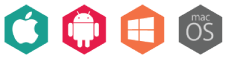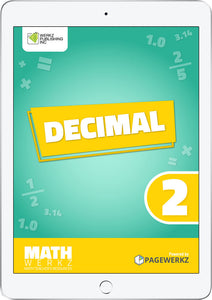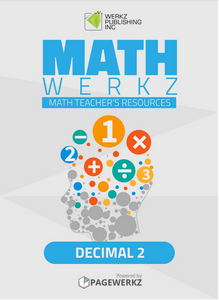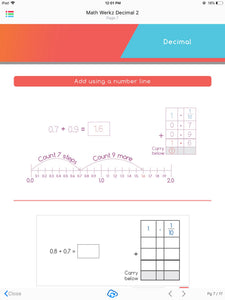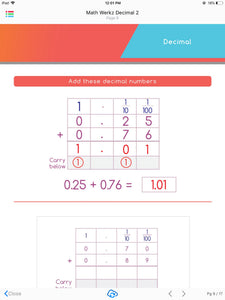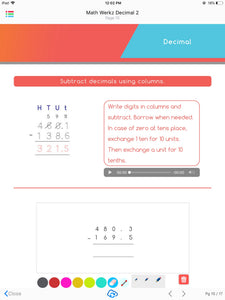# Math Werkz Decimal 2

Regular price \$2.00 Sale

Product Description:

Math Werkz Decimal 2 is a fun, interactive courseware that teaches kids about decimals.

Intended to discuss decimal numbers and fractions sums, this courseware includes worksheets and activities that may help kids in learning more about adding, subtracting, and rounding off decimal numbers. Aligned with the Common Core State Standards Initiative (CCSI) for fifth-graders, among the activities included are adding decimal numbers using a number line, mental subtraction trick, subtracting numbers without borrowing, finding the missing numbers, and subtracting decimals using columns. Teachers can also use this as additional exercises or classroom-based activities for their students to work on.

Math Werkz Decimal 2 is one of four Math Werkz Decimal interactive eBooks.

Math Werkz Series is a Primary Mathematics Teacher Resource consisting of 90+ activity-based worksheets. These worksheets have teacher-enabled tools that can be used to teach and engage students using any available devices like tablets, iPads, smartphones, or classroom devices like whiteboards, SMART Boards or PCs.

Overall, there is a total of 98 Math Werkz interactive eBooks available with topics that range from Factors & Multiples, Fraction, Money, Time, Division, Geometry, Measurement, Graphs and Charts, Multiplication, Percentage, Decimal, Area and Perimeter. Assessment Book, Addition, Subtraction and Number System.

Author: Werkz Publishing Inc.

Number of pages: 17

Available platforms: